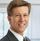## MidPoint: the half-way point along a great circle path between two points

Started ‎04-11-2021 by
Modified ‎04-13-2021 by
Views 4,196

Base SAS has some GIS-type functions (GIS = Geographic Information System), the most useful one is beyond any doubt the GEODIST function that returns the geodetic distance between two latitude and longitude coordinates.

Remember that Euclidean distances won’t work on earth or any other (near-)spherical surface. On earth you need the geodesic distance (the great-circle distance). See this article about how to calculate geodesic distance using GEODIST or PROC DISTANCE.

However, there is no function for the MIDPOINT which is the half-way point along a great circle path between two points / positions. I present a little program below to calculate (the latitude-longitude coordinates of) this midpoint. The formula used is for calculating the midpoint based on a spherical earth (ignoring ellipsoidal effects) – which is accurate enough for most purposes… It’s hence a formula from spherical trigonometry.

This program can be useful for geographers, transport economists, transport scientists, shipping companies, airline companies and the like.

The program is using positions of two objects; objects can be cars, vessels (ships), airplanes, persons, …

``````PROC DATASETS library=work nolist;
delete positions_two_objects_in_degrees / memtype=DATA; run;
delete DS_with_all_variables            / memtype=DATA; run;
QUIT;

data work.positions_two_objects_in_degrees;
latitude1=80; longitude1=-121; latitude2=-80; longitude2=23; output;
latitude1=45; longitude1=-175; latitude2=-80; longitude2=70; output;
run;

/* conversion from degrees to radians                                         */
/* The reason for converting degrees to radians in below program is           */
/* because the COS and SIN functions require an argument specified in radians */
/* and, also, the ATAN2 returns the result in radians                         */
set work.positions_two_objects_in_degrees;
array degrees{4} latitude1 longitude1 latitude2 longitude2;
pi = constant('pi');
do i = 1 to dim(degrees);
end;
run;

/* conversion from miles to nautical miles:                             */
/* for an approximate result, divide the length value in miles by 1,151 */
run;

/* What are the coordinates (latitude, longitude) of the Midpoint?:             */
/* This is the half-way point along a great circle path between the two points. */

/* FOR GEODIST :                                                                      */
/* If the LATITUDE  value is expressed in degrees, it must be between 90   and –90.   */
/* If the LATITUDE  value is expressed in radians, it must be between pi/2 and –pi/2. */
/* If the LONGITUDE value is expressed in degrees, it must be between 180  and –180.  */
/* If the LONGITUDE value is expressed in radians, it must be between pi   and –pi.   */
latRMid =max(-pi/2,latRMid) ; latRMid =min(pi/2,latRMid) ;
longRMid=max(-pi  ,longRMid); longRMid=min(pi  ,longRMid);

latDMid =(latRMid *180)/pi; /* conversion from radians to degrees */
longDMid=(longRMid*180)/pi; /* conversion from radians to degrees */
run;

data work.DS_with_all_variables(replace=yes repempty=no);
LENGTH result \$ 7;
if abs(Distance_Position1_to_MidPoint-Distance_Position2_to_MidPoint)/Distance > 0.025
then result='WRONG';
else result='CORRECT';
run;

PROC DATASETS library=work nolist;
modify DS_with_all_variables (label='Data set with coordinates and midpoint and distances');
label result     = 'midpoint formula seems CORRECT (wanted) or WRONG (not wanted)';
label latitude1  = 'latitude  of object_1 in degrees';
label longitude1 = 'longitude of object_1 in degrees';
label latitude2  = 'latitude  of object_2 in degrees';
label longitude2 = 'longitude of object_2 in degrees';
label latRMid    = 'latitude  of the midpoint in radians';
label longRMid   = 'longitude of the midpoint in radians';
label latDMid    = 'latitude  of the midpoint in degrees';
label longDMid   = 'longitude of the midpoint in degrees';
label Distance   = 'distance between both objects in nautical miles';
label Distance_Position1_to_MidPoint = 'distance between object_1 and MidPoint in nautical miles';
label Distance_Position2_to_MidPoint = 'distance between object_2 and MidPoint in nautical miles';
label pi = 'the constant pi (the ratio of the circumference of any circle to the diameter of that circle)';
run;
QUIT;
/* end of program */``````

SAS has a number of other features to support mapping and analytics related to geospatial data:

Enjoy reading and who knows, it may come in handy one day.It seems like you can generalize this to produce a fraction f in [0, 1] along the great circle from p1 to p2. Your formula is the special case for f=0.5.

Version history
Last update:
‎04-13-2021 08:28 AM
Updated by:Contributors
Article Labels
Article Tags